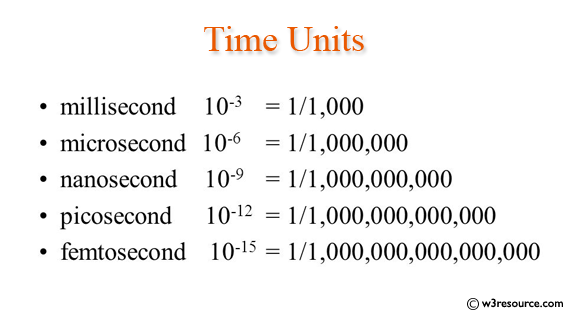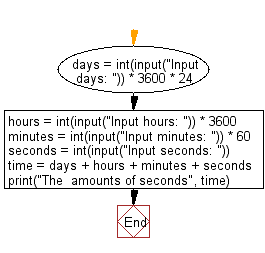﻿ Python: Convert all units of time into seconds - w3resource# Python: Convert all units of time into seconds

## Python Basic: Exercise-62 with Solution

Write a Python program to convert all units of time into seconds.

Pictorial Presentation:Sample Solution:-

Python Code:

``````days = int(input("Input days: ")) * 3600 * 24
hours = int(input("Input hours: ")) * 3600
minutes = int(input("Input minutes: ")) * 60
seconds = int(input("Input seconds: "))

time = days + hours + minutes + seconds

print("The  amounts of seconds", time)
```
```

Sample Output:

```Input days:  4
Input hours:  5
Input minutes:  20
Input seconds:  10
The  amounts of seconds 364810
```

Flowchart:## Visualize Python code execution:

The following tool visualize what the computer is doing step-by-step as it executes the said program:

Python Code Editor:

Have another way to solve this solution? Contribute your code (and comments) through Disqus.

What is the difficulty level of this exercise?

Test your Python skills with w3resource's quiz

﻿

## Python: Tips of the Day

Try-catch-else construct:

```try:
foo()
except Exception:
print("Exception occured")
else:
print("Exception didnt occur")
finally:
print("Always gets here")
```